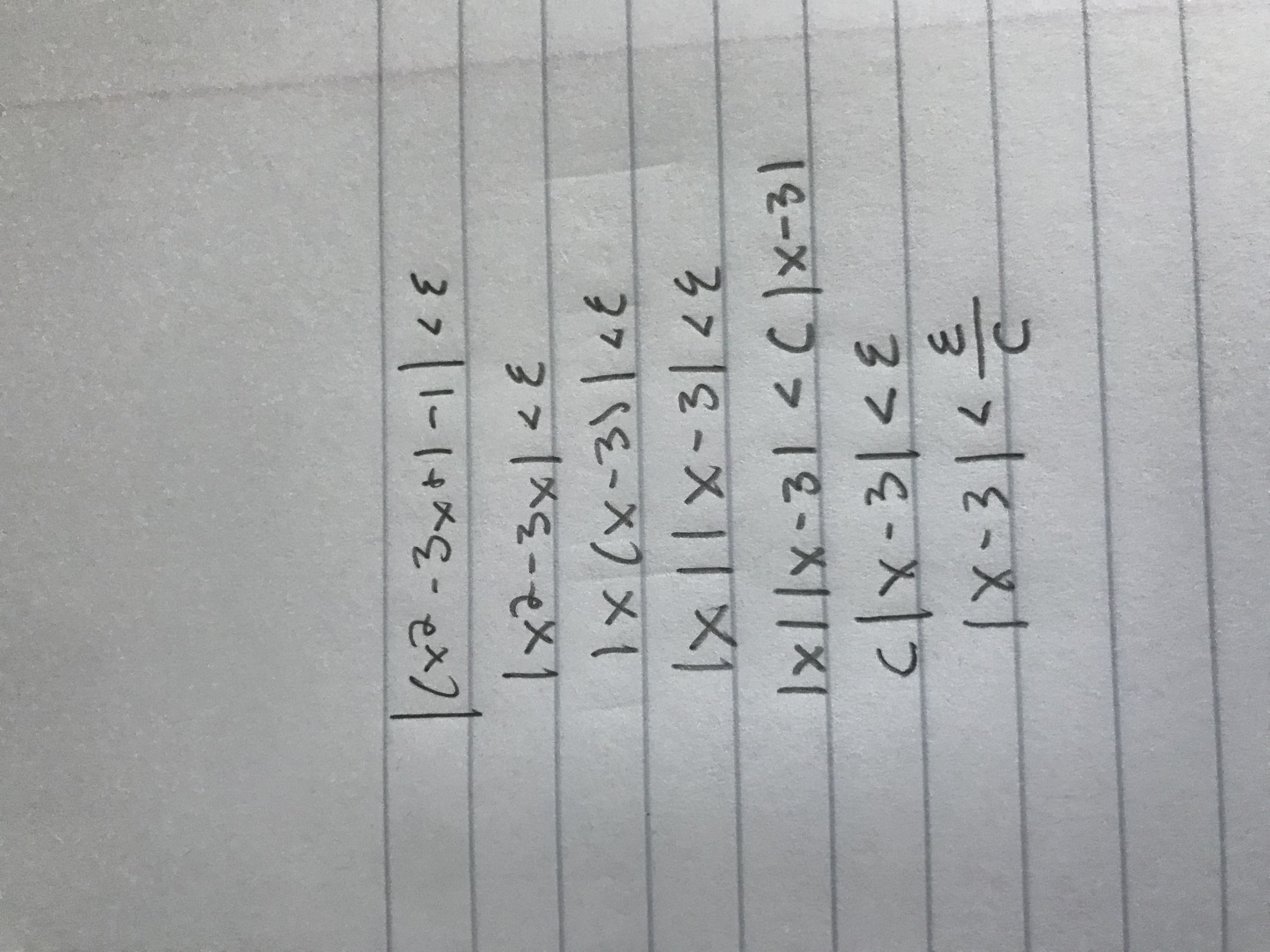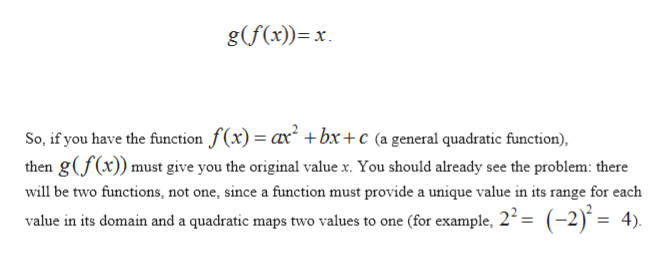|২ -3x+] -|

Question

Hi, I'm struggling to understand this whole problem. I did attempt to do #4 (not sure if it's right?), but as for #1-3 I have no idea where to even start. Thank you for your help!

This is the problems:

Let y(x) = ax2 + bx + c. The function y(x) is not invertible, however, like the function f(x) = x2 we can make some restrictions on its domain to make it invertible. Hint: Think of the example (x2) given above.

• Find the largest possible domain where y(x) is invertible
• Find its inverse function using this domain
• Check that it is indeed the inverse function by verifying the identity y−1(y(x)) =y(y−1(x)) = x
• Show limx→3 x2 −3x+1 = 1 using the definition of limit, that is, using ε and δ.help_outlineImage Transcriptionclose|২ -3x+] -| <६ |x-১y| < ४. 7|९६-X) x { |x || x-5| <१ १-X lxLlx-31 C1x-31 ६ clx-312 |x-३|८- C कैहहा fullscreen
Step 1

Given function is

Step 2

Invertible function: a function is said to be an invertible function if inverse of that function is also a function (one to one relationship)

Step 3

the inverse of a function f is a functio...help_outlineImage Transcriptioncloseg(f(x)) x So, if you have the function f(x) ax +bx +c (a general quadratic function), then gf(x)) must give you the original value x. You should already see the problem: there will be two functions, not one, since a funetion must provide a unique value in its range for each value in its domain and a quadratic maps two values to one (for example, 22= (-2) = 4) fullscreen

Want to see the full answer?

See Solution

Want to see this answer and more?

Our solutions are written by experts, many with advanced degrees, and available 24/7

See Solution
Tagged in

Functions